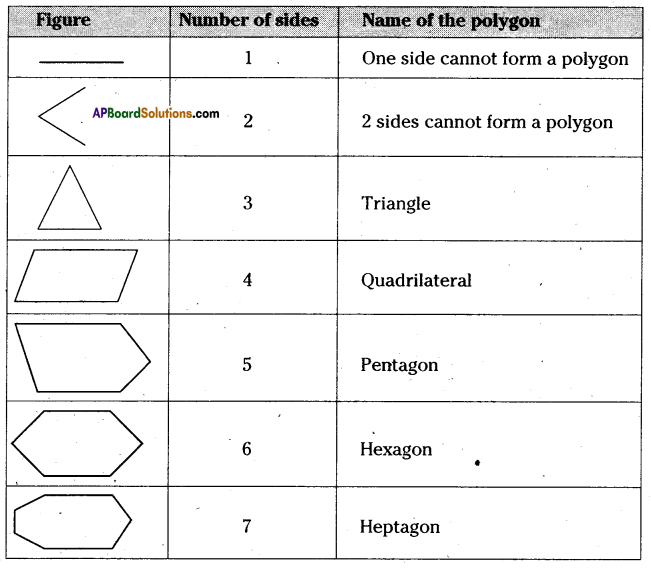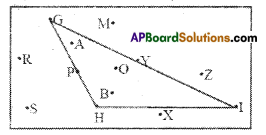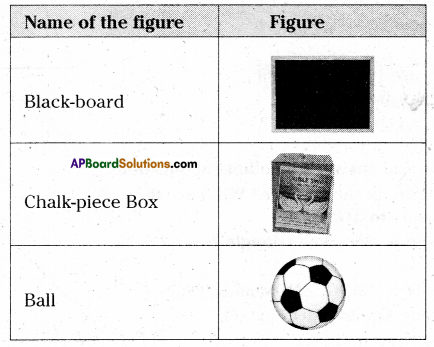AP State Syllabus AP Board 6th Class Maths Solutions Chapter 9 2D-3D Shapes InText Questions and Answers.

## AP State Syllabus 6th Class Maths Solutions 9th Lesson 2D-3D Shapes InText Questions(Page No. 125)

Question 1.
Draw six different types of rough sketches of polygons in your notebook. In which case, it is not possible to form a polygon ?
Hence, what is the least number of sides needed to form a polygon ? Obviously three.
Solution:From the above figure we conclude that with one side and two sides cannot form a polygon. So, atleast three sides needed to form a polygon.Check Your Progress (Page No. 127)Question 1.
Which points are marked in the interior of A GHI ?
Solution:
The points marked in the interior of AGHI are A, B and 0.

Question 2.
Which points sure marked on the triangle?
Solution:
The points marked on the triangle are G, P, H, I and Y.

Question 3.
Which points are’marked in the exterior of A GHI ?
Solution:
The points marked in the exterior of AGHI are M, R, S, X and Z.

(Page No. 130)

Question 1.
Take a rectangular sheet (like a post-card). Fold it along its length so that one half fits exactly over the other half. Is this fold a line of symmetry ? Why ?
Solution:
Yes. Because the two parts of the rectangular sheet (post-card) coincide exactly with each other.
So, the folded line is the line of symmetry.Question 2.
Open it up now and again fold along its width in the same way. Is this second fold also a line of symmetry ? Why ?
Solution:
Yes. Because the two parts of the rectangular sheet (post-card) coincide exactly with each other.
So, the second folded line also a line of symmetry.

Question 3.
Do you find that these two lines are the lines of symmetry ?
Solution:
Yes. These two lines are the lines of symmetry. One is line of symmetry along length and the other is line of symmetry along width.

Project (Page No. 130)

Question 1.
Collect symmetrical figures from your surroundings and prepare a scrap book.
Solution: